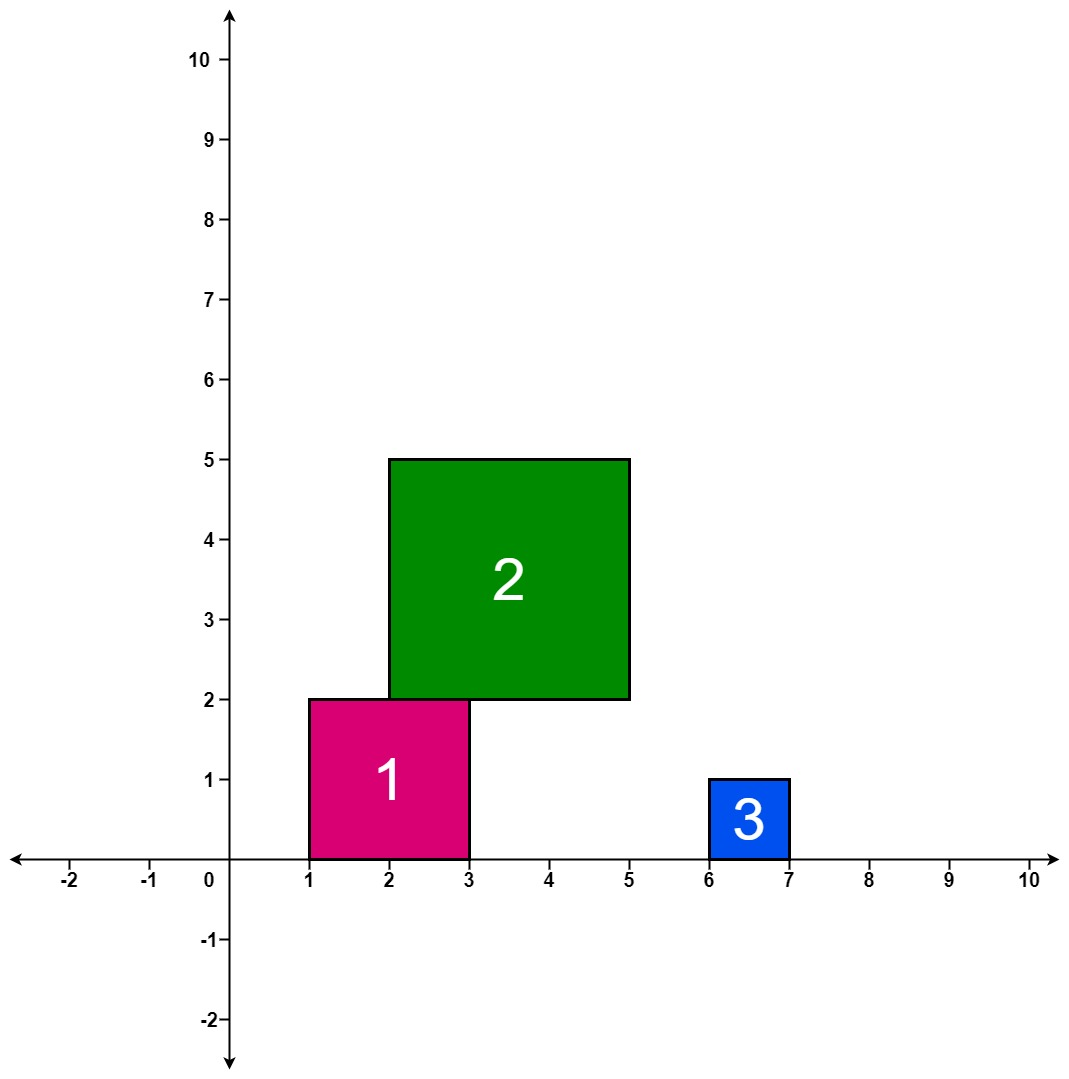## Algorithm

Problem Name: 699. Falling Squares

There are several squares being dropped onto the X-axis of a 2D plane.

You are given a 2D integer array `positions` where `positions[i] = [lefti, sideLengthi]` represents the `ith` square with a side length of `sideLengthi` that is dropped with its left edge aligned with X-coordinate `lefti`.

Each square is dropped one at a time from a height above any landed squares. It then falls downward (negative Y direction) until it either lands on the top side of another square or on the X-axis. A square brushing the left/right side of another square does not count as landing on it. Once it lands, it freezes in place and cannot be moved.

After each square is dropped, you must record the height of the current tallest stack of squares.

Return an integer array `ans` where `ans[i]` represents the height described above after dropping the `ith` square.

Example 1:```Input: positions = [[1,2],[2,3],[6,1]]
Output: [2,5,5]
Explanation:
After the first drop, the tallest stack is square 1 with a height of 2.
After the second drop, the tallest stack is squares 1 and 2 with a height of 5.
After the third drop, the tallest stack is still squares 1 and 2 with a height of 5.
Thus, we return an answer of [2, 5, 5].
```

Example 2:

```Input: positions = [[100,100],[200,100]]
Output: [100,100]
Explanation:
After the first drop, the tallest stack is square 1 with a height of 100.
After the second drop, the tallest stack is either square 1 or square 2, both with heights of 100.
Thus, we return an answer of [100, 100].
Note that square 2 only brushes the right side of square 1, which does not count as landing on it.
```

Constraints:

• `1 <= positions.length <= 1000`
• `1 <= lefti <= 108`
• `1 <= sideLengthi <= 106`

## Code Examples

### #1 Code Example with Javascript Programming

```Code - Javascript Programming```

``````
class Interval {
constructor(start, end, height) {
this.start = start
this.end = end
this.height = height
}
}
function fallingSquares(positions) {
const intervals = []
const res = []
let h = 0
for (let pos of positions) {
let cur = new Interval(pos, pos + pos, pos)
h = Math.max(h, getHeight(intervals, cur))
res.push(h)
}
console.log(intervals)
return res
}
function getHeight(intervals, cur) {
let preMaxHeight = 0
for (let i of intervals) {
if (i.end <= cur.start) continue
if (i.start >= cur.end) continue
preMaxHeight = Math.max(preMaxHeight, i.height)
}
cur.height += preMaxHeight
intervals.push(cur)
return cur.height
}
``````
Copy The Code &

Input

cmd
positions = [[1,2],[2,3],[6,1]

Output

cmd
[2,5,5]

### #2 Code Example with Python Programming

```Code - Python Programming```

``````
class Solution:
def fallingSquares(self, positions: List[List[int]]) -> List[int]:
height = 
pos = 
res = []
max_h = 0
for left, side in positions:
i = bisect.bisect_right(pos, left)
j = bisect.bisect_left(pos, left + side)
high = max(height[i - 1:j] or ) + side
pos[i:j] = [left, left + side]
height[i:j] = [high, height[j - 1]]
max_h = max(max_h, high)
res.append(max_h)
return res
``````
Copy The Code &

Input

cmd
positions = [[1,2],[2,3],[6,1]

Output

cmd
[2,5,5]